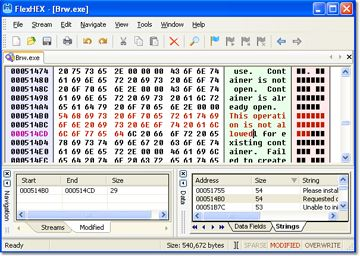# 0xFF...FF / 10 == 0x19...99A few weeks ago, I wrote a C++ routine to parse decimal numbers using the overflow detection principles of SafeInt. I couldn’t find anything in the libraries that actually did a good job of checking for overflow.

Briefly, to see if unsigned values A+B overflow, check if (A > MAX_UINT - B). Similarly, A*B will overflow if (A > MAX_UINT / B).

```// Convert a string to an unsigned. Returns 'true' iff conversion is legitimate.
bool
StringToUnsigned(
const string& str,
unsigned&     rUint)
{
rUint = 0;

if (str.empty())
return false;

for (unsigned i = 0;  i < str.length();  ++i)
{
if (!isdigit(str[i]))
return false;

// Check for numeric overflow.
if (rUint > numeric_limits<unsigned>::max() / 10)
return false;
rUint *= 10;

unsigned d = str[i] - '0';
if (rUint > numeric_limits<unsigned>::max() - d)
return false;
rUint += d;
}

return true;
}
```

While debugging this code, I noticed something in­ter­est­ing. 0xFFFFFFFF divided by ten (0xA) is 0x19999999. This pattern holds for smaller and larger sequences of 0xFF…FF too: 0xFF/10 = 0x19; 0xFFFF/10 = 0x1999; and so on.

I’m not sure how to prove this, but I can prove the closely related result: 0x19…99 * 10 = 0xFF…FA:

```10 * N         = 8 * N  +  2 * N
10 * 0x19...99 = 8 * 0x19...99  +  2 * 0x19...99

0x199...99     =   %0001 1001 1001 ... 1001 1001

10 * 0x19...99 =   %1100 1100 1100 ... 1100 1000
+ %0011 0011 0011 ... 0011 0010
=   %1111 1111 1111 ... 1111 1010
```

A mildly curious result of no value, but it amused me.